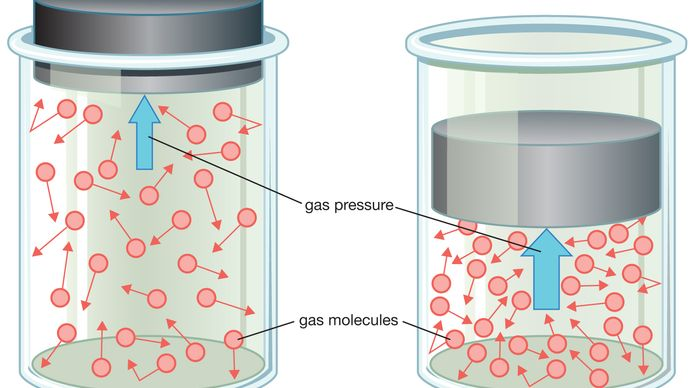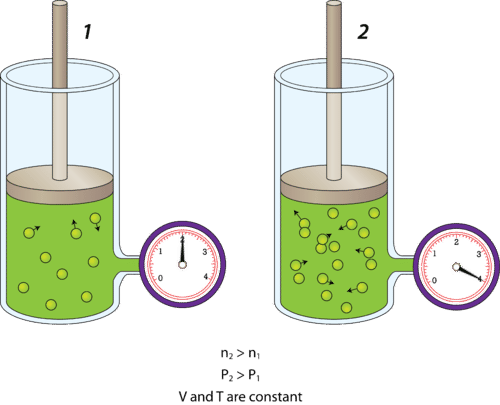# Gas Pressure Study Guide

What’s the definition of gas pressure? In general, pressure is defined as the force exerted per unit of area. In the case of a gas, pressure is the force exerted by the gaseous molecules on the vessel that holds them. Gas particles move quickly and randomly in straight lines, colliding with both each other and the walls of their container. The greater the number of collisions that occur with the container walls, the higher the pressure of the gas.### WHAT IS THE GAS PRESSURE FORMULA?

We can find the pressure of a gas using either of the following formulas:

P = F/A

P = pressure (Pa)

A= area (m²)

F = impact force due of gaseous molecule collisions (N)

P= nRT/V

V = volume (m³)

n = number of gas particles (mol)

R = universal gas constant (8.31446261815324 m³⋅Pa⋅K⁻¹⋅mol⁻¹)

T = temperature (K)

### FACTORS THAT AFFECT GAS PRESSURE

The number of gas particles (n): Gas pressure is affected by the number of gas particles present within the vessel that holds them. Imagine you have a rigid container with a fixed volume, temperature, and amount of gas. This container will have some particular pressure according to these variables. As we know, gas pressure results from the number of collisions that occur between gas particles and the walls of their container. If we add more gas particles to the container, the number of collisions, and thus the pressure, will increase. In the reverse, the removal of gas particles will decrease the gas pressure within the container.Volume (V): Gas pressure is affected by the volume of its container. If we take the gas from the previous example and place it in a container with a lesser volume, the same number of gas particles will have less space in which to move around. As a result, the number of collisions will increase, and so will the gas pressure.

Temperature (T): Temperature is related to gas pressure based on the kinetic molecular theory. If you increase the temperature of a container of gas, the particles inside will gain kinetic energy from the heat. The higher the kinetic energy of a particle, the faster it moves. Thus, increased temperature results in more collisions and consequently a higher pressure.

### CONCLUSION

• Gas pressure is the force exerted by gaseous molecules as they collide with the walls of their container.

• If the volume and temperature of a container of gas are held constant, an increase in the number of gas particles will result in an increase in pressure, and vice versa.

• If the temperature and number of particles of a container of gas are held constant, an increase in volume will result in a decrease in pressure, and vice versa.

• If the volume and number of gas particles are held constant, an increase in temperature will result in an increase in pressure, and vice versa.

• An increase in temperature results in an increased speed of gas particle movement, and thus a higher pressure. , the pressure of the gas within the container would increase too. A decrease in the volume of the container results in increased pressure.

• Gas pressure can be determined using the following equations:

P = F/A

P = pressure (Pa)

A = area (m²)

F = impact force due of gaseous molecule collisions (N)

P= nRT/V

V = volume (m³)

n = number of gas particles (mol)

R = universal gas constant (8.31446261815324 m³⋅Pa⋅K⁻¹⋅mol⁻¹)

T = temperature (K)

### FAQs

1. What formulas can be used to calculate gas pressure?

P= F/A or P = nRT/V.

2. What is gas pressure?

Gas pressure is the force exerted by gaseous molecules colliding with the walls of their container.

We hope you enjoyed studying this lesson and learned something cool about Gaseous Pressure! Join our Discord community to get any questions you may have answered and to engage with other students just like you! Don’t forget to download our App to experience our fun VR classrooms – we promise, it makes studying much more fun! 😎

]]>